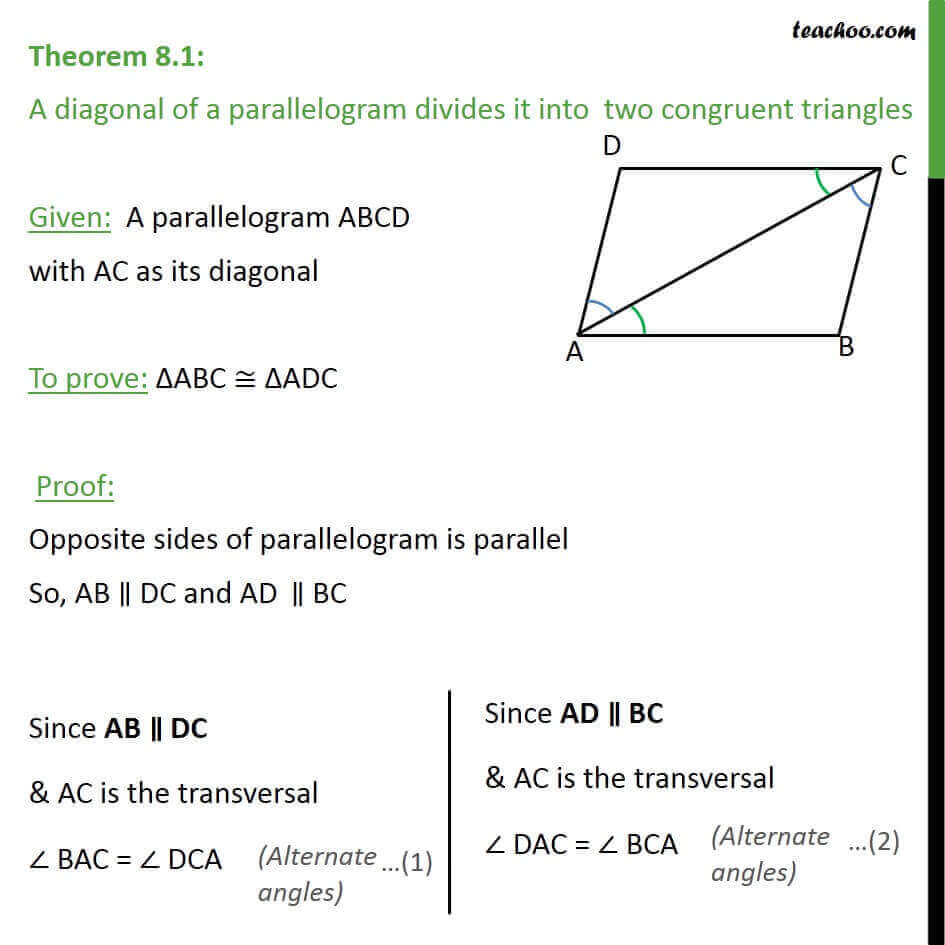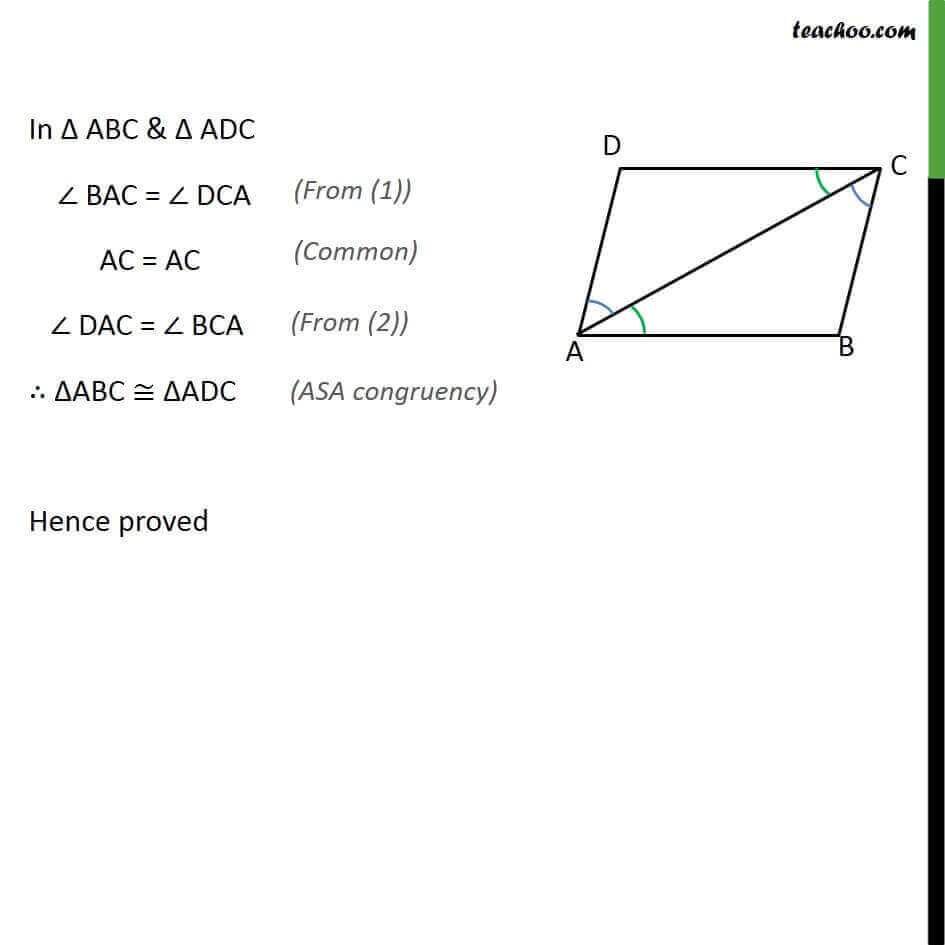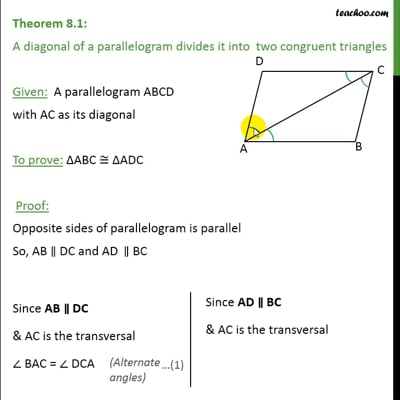Theorems

Serial order wiseThis video is only available for Teachoo black users

Learn in your speed, with individual attention - Teachoo Maths 1-on-1 Class

### Transcript

Theorem 8.1: A diagonal of a parallelogram divides it into two congruent triangles Given: A parallelogram ABCD with AC as its diagonal To prove: ΔABC ≅ ΔADC Proof: Opposite sides of parallelogram is parallel So, AB ∥ DC and AD‖∥ BC In Δ ABC & Δ ADC ∠ BAC = ∠ DCA AC = AC ∠ DAC = ∠ BCA ∴ ΔABC ≅ ΔADC Hence proved